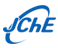SCI和EI收录∣中国化工学会会刊

Chinese Journal of Chemical Engineering ›› 2019, Vol. 27 ›› Issue (3): 483-500.

• Reviews •

### Quadrature-based moment methods for the population balance equation: An algorithm review

Dongyue Li1,2, Zhipeng Li1, Zhengming Gao1

1. 1 State Key Laboratory of Chemical Resource Engineering, School of Chemical Engineering, Beijing University of Chemical Technology, Beijing 100029, China;
2 State Key Laboratory of Advanced Metallurgy, University of Science and Technology Beijing, Beijing 100083, China
• Received:2018-03-21 Revised:2018-10-04 Online:2019-04-25 Published:2019-03-28
• Contact: Zhengming Gao,E-mail address:gaozm@mail.buct.edu.cn

### Quadrature-based moment methods for the population balance equation: An algorithm review

Dongyue Li1,2, Zhipeng Li1, Zhengming Gao1

1. 1 State Key Laboratory of Chemical Resource Engineering, School of Chemical Engineering, Beijing University of Chemical Technology, Beijing 100029, China;
2 State Key Laboratory of Advanced Metallurgy, University of Science and Technology Beijing, Beijing 100083, China
• 通讯作者: Zhengming Gao,E-mail address:gaozm@mail.buct.edu.cn

Abstract: The dispersed phase in multiphase flows can be modeled by the population balance model (PBM). A typical population balance equation (PBE) contains terms for spatial transport, loss/growth and breakage/coalescence source terms. The equation is therefore quite complex and difficult to solve analytically or numerically. The quadrature-based moment methods (QBMMs) are a class of methods that solve the PBE by converting the transport equation of the number density function (NDF) into moment transport equations. The unknown source terms are closed by numerical quadrature. Over the years, many QBMMs have been developed for different problems, such as the quadrature method of moments (QMOM), direct quadrature method of moments (DQMOM), extended quadrature method of moments (EQMOM), conditional quadrature method of moments (CQMOM), extended conditional quadrature method of moments (ECQMOM) and hyperbolic quadrature method of moments (HyQMOM). In this paper, we present a comprehensive algorithm review of these QBMMs. The mathematical equations for spatially homogeneous systems with first-order point processes and second-order point processes are derived in detail. The algorithms are further extended to the inhomogeneous system for multiphase flows, in which the computational fluid dynamics (CFD) can be coupled with the PBE. The physical limitations and the challenging numerical problems of these QBMMs are discussed. Possible solutions are also summarized.